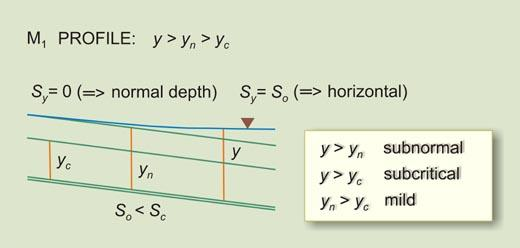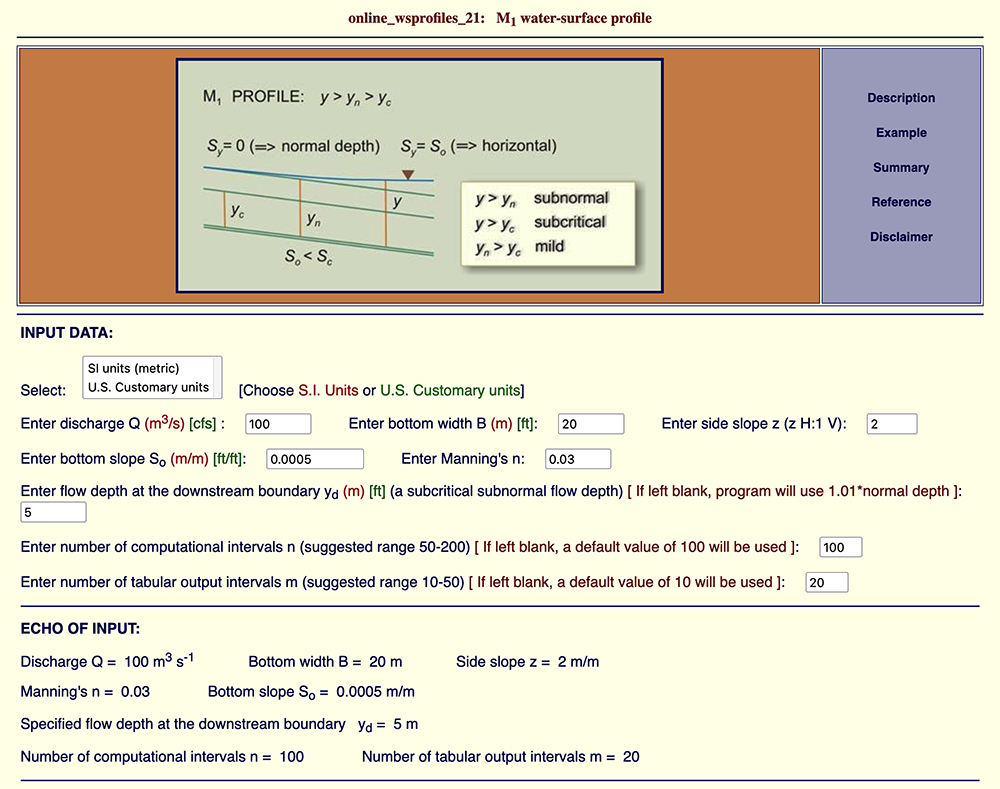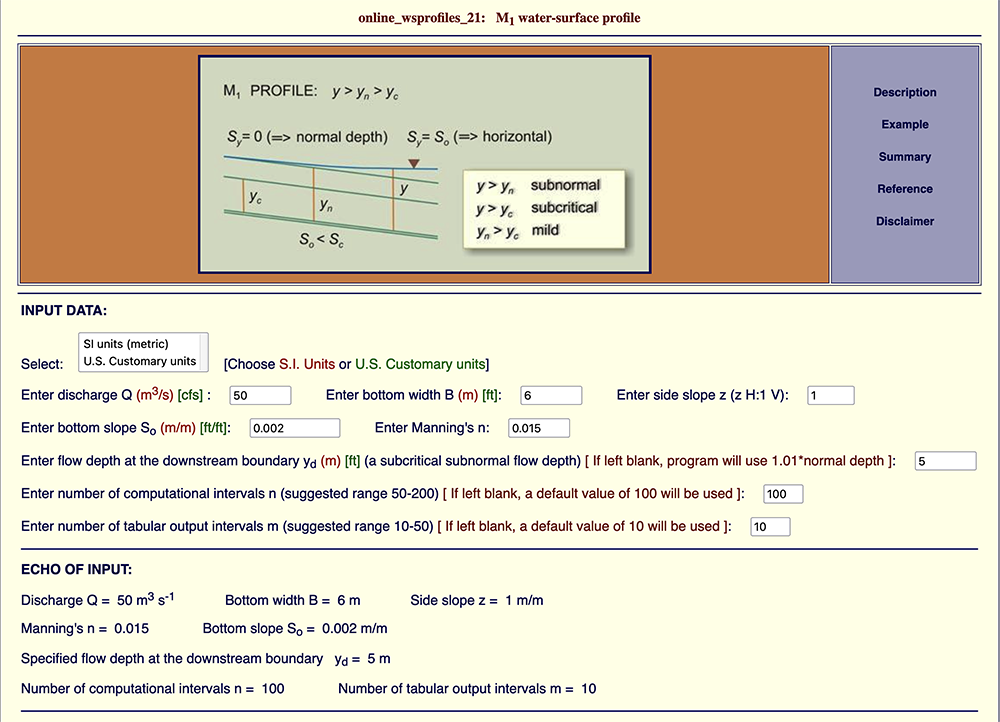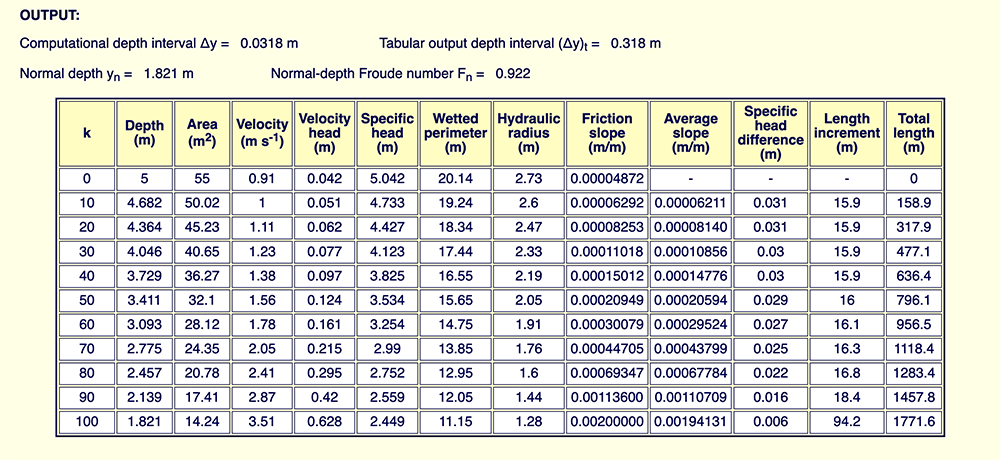M1 water-surface profile.

M1 WATER-SURFACE PROFILE CALCULATED ONLINE

Victor M. Ponce

Professor of Civil and Environmental Engineering

San Diego State University, San Diego, California

10 November 2023

 ABSTRACT. An online calculation of an M1 open-channel flow water-surface profile is shown in detail. Two examples using the script ONLINE_WSPROFILES_21 demonstrate the utility of using this online digital tool for the accurate and expedient calculation of an M1 water-surface profile.

1.  INTRODUCTION

A water-surface profile is a feature of the hydraulics of open channels which describes the variation of the water-surface elevation in the longitudinal direction (one dimension x in space), under a steady equilibrium flow condition. There are twelve (12) types of water-surface profiles, depending on the Froude number F and the ratio So /Sc , in which So = bottom slope, and Sc = critical slope. The critical slope Sc is equal to 1/8 of the Darcy-Weisbach friction factor f. Table 1 lists the twelve types of profiles (Ponce, 2014).

 Table 1.  The twelve (12) types of water-surface profiles. Family Character Rule So > Sc So = Sc So < Sc So = 0 So < 0 I Retarded(Backwater) 1 > F 2 < (So / Sc) S1 C1 M1 - - II A Accelerated(Drawdown) 1 < F 2 < (So / Sc) S2 - - - - B Accelerated(Drawdown) 1 > F 2 > (So / Sc) - - M2 H2 A2 III Retarded(Backwater) 1 < F 2 > (So / Sc) S3 C3 M3 H3 A3

In this article, we describe the M1 water-surface profile, a retarded (backwater) subcritical/subnormal profile, which may well be the most common profile in practice. The M1 profile depicts flow in a mild channel, upstream of a reservoir (Fig. 1). We present two examples and show the respective online calculations.Fig. 1  M1 water-surface profile.

2.  GOVERNING EQUATION

Chow (1959) has presented the classical way of expressing the governing equation of steady, gradually varied flow. A more cogent, dimensionless presentation, focusing on critical slope, has been advanced by Ponce (2014) and is presented here.

In terms of critical slope, the general equation for flow-depth gradient dy/dx is:

 dy           So  -  (P / T ) (Tc / Pc ) Sc F 2               _____  =  ________________________________   dx                            1  -  F 2 (1)

in which So = channel (bottom) slope, Sc = critical slope, P = wetted perimeter, T = channel top width, Tc = channel top width at critical flow, Pc = wetted perimeter at critical flow, and F = Froude number. The Froude number is defined as follows: F = v/(gD)1/2, in which v = mean flow velocity, g = gravitational acceleration, and D = hydraulic depth, in which D = A /T.

For (P / T )  =  (Pc / Tc ), Eq. 1 reduces to:

 dy           So  -  Sc F 2               _____  =  _______________   dx               1  -  F 2 (2)

For conciseness, the flow-depth gradient may be written as:

 dy                          Sy  =  _____             dx (3)

Substituting Eq. 3 into Eq. 2, the flow-depth gradient is:

 Sy           (So / Sc)  -  F 2       ____  =  __________________  Sc                 1  -  F 2 (4)

Equation 2, or Equation 4, its reduced form, is the steady gradually varied flow equation (Fig. 2). The depth gradient Sy is shown to be a function only of the following variables: (1) channel (bottom) slope So, (2) critical slope Sc , and (3) Froude number F.Fig. 2  Definition sketch for energy balance in open-channel flow.

3.  ONLINE CALCULATION:  EXAMPLE A

We pose an example of the calculation of an M1 profile in a natural channel using the online calculator ONLINE_WSPROFILES_21. The following box shows the input data.

 Example A:  Input Data Discharge Q = 100 m3/s Bottom width = 20 m Side slope z = 2 (z H : 1 V) Bottom slope So = 0.0005 Manning's n = 0.03 Normal depth at the downstream boundary yd = 5 m Number of computation intervals: n = 100 Number of tabular output intervals: m = 20

 [Click on top of figure to expand]Fig. 3 (a)  Example A:  Input.

 [Click on top of figure to expand]Fig. 3 (b)  Example A:  Output.

Output.  The results show that the (flow) depth at the downstream boundary (yd = 5 m) will decrease gradually to the normal depth in the upstream end yn = 2.962 m. The total distance, from downstream boundary to upstream end, is: L = 13,114.5 m.

4.  ONLINE CALCULATION:  EXAMPLE B

We pose an example of the calculation of an M1 profile in a lined channel using the online calculator ONLINE_WSPROFILES_21. The following box shows the input data.

 Example B:  Input Data Discharge Q = 50 m3/s Bottom width = 6 m Side slope z = 1 (z H : 1 V) Bottom slope So = 0.002 Manning's n = 0.015 Normal depth at the downstream boundary yd = 5 m Number of computation intervals: n = 100 Number of tabular output intervals: m = 10

 [Click on top of figure to expand]Fig. 4 (a)  Example B:  Input.

 [Click on top of figure to expand]Fig. 4 (b)  Example B:  Output.

Output.  The results show that the (flow) depth at the downstream boundary (yd = 5 m) will decrease gradually to the normal depth in the upstream end yn = 1.821 m. The total distance, from downstream boundary to upstream end, is: L = 1,771.6 m.

5.  SUMMARY

An online calculation of an M1 open-channel flow water-surface profile is shown in detail. Two examples using the script ONLINE_WSPROFILES_21 demonstrate the utility of using this online digital tool for the accurate and expedient calculation of an M1 water-surface profile.

REFERENCES

Chow, V. T. 1959. Open-channel hydraulics. McGraw-Hill, Inc, New York, NY.

Ponce, V. M. 2014b. Fundamentals of Open-channel Hydraulics. Online textbook.
https://ponce.sdsu.edu/openchannel/index.html

 231025